## Week 3 Coding Lecture 1: Linear Systems

Below we include a recap of the theory for convenience, but in this lecture we will skip to the coding part. For the theoretical details please see the theory lecture.

### Matrix multiplication

Remember from the first week of class that we have defined two different ways to multiply matrices. As an example, suppose that we have the two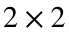matricesand.
In MATLAB we would write this as:
A = [1 2; 3 4];
B = [-2 1; 0 3];
We have defined the elementwise multiplication of A and B as,
or in MATLAB:
C = A .* B
C = 2×2
-2 2 0 12
More generally, as long as A and B are exactly the same size and shape, we define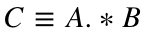by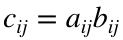. That is, the entry in the ith row, jth column of C is the product of the entry in the ith row, jth column of A and the entry in the ith row, jth column of B.
In MATLAB, this means that
C(i, j) = A(i, j) * B(i, j);
for any valid row/column indices i and j.
We also defined normal (linear algebra) multiplication of A and B as,
or in MATLAB:
D = A * B
D = 2×2
-2 7 -6 15
More generally, as long as the number of columns of A is the same as the number of rows of B, we define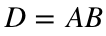as the matrix where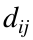(the entry in the ith row, jth column) is the dot product of the ith row of A with the jth column of B. If n is the number of columns of A and the number of rows of B, then we can write this as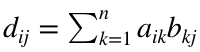. You should confirm to yourself that this is equivalent to the dot product definition.
If you are not familiar with linear algebra, then this second definition probably seems a little strange. As we will see in a moment, the big advantage of this definition is that it makes it easy to write linear systems of equations.

### Systems of equations

One of the most important skills we will cover in this class is how to efficiently solve systems of linear equations. It turns out that such systems arise when solving a wide variety of problems in applied mathematics, even if the original problem does not appear linear. What do we mean by a system of linear equations? You should already be familiar with the concept from a basic algebra class. For instance:or(If we only have two variables, we will often call them x and y. If there are three variables, we will sometimes call them x, y and z. For anything more complicated, we will use a single letter with different subscripts like in the second example.) For starters, let's focus on the first pair of equations. We have two equations and two variables, so we refer to this as a(read: two by two) system. The first number indicates the number of equations and the second number indicates the number of variables. You have probably already seen several ways to solve this system.

#### Substitution

One approach would be to solve the first equation for y, then substitute this into the second equation and solve for x. Solving the first equation for y gives us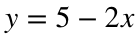. Substituting that into the second equation gives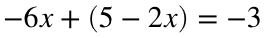, so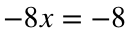and therefore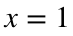.
Plugging this back intogives us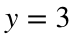. The final solution to the system is therefore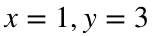.
This method is called substitution. It is an easy approach when we only have a couple of equations, but it rapidly becomes messy when we try to generalize to larger systems. We will therefore only use substitution in certain special cases.

#### Elimination

You have probably seen another approach, where we try to cancel out one of the variables from one of the equations by adding/subtracting different equations. For example, if we multiplied the first equation by 3 and then added it to the second equation, we would cancel out the x's. We then obtainWe denote this step by: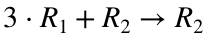You should read this as "multiply row 1 by 3 and add it to row 2, then change the second row to this result."
This process is called elimination. Notice that we have not solved the system yet, but we did make it much easier to solve by substitution. The second equation is now very easy to solve for y, and we find that. Now that we have this value, we can substitute it into the first equation to find that. The system we obtained from elimination is called upper triangular because the nonzero coefficients form a triangle in the upper right (and the zero coefficients are all in the lower left). When we use the substitution method on an upper triangular system, it is called back substitution (because we find the variables in backwards order).
Note that it would be just as easy to make the coefficient of y in the first equation zero. This would give us a system where all the nonzero coefficients are in the lower left corner and all the zero coefficients are in the upper right. Such a system is called lower triangular and we could solve it in the opposite order with forward substitution. Either method would work equally well. In general, we call these systems triangular.
This method generalizes much better when we start solving larger systems. For example, to solve the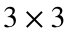system given at the beginning of this section, we want to eliminate (i.e., turn to zero) all the coefficients in the lower left. That is, we want to make a system of the formOnce we do this, it will be easy to solve the resulting system with back substitution. There are several approaches to this problem, but if you are not systematic then it is easy to accidentally undo your work. We will therefore always follow a standard order. This is easier to see with an example.
First, we will eliminate the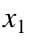coefficient from the second equation. We can do this by multiplying the first equation by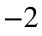and adding it to the second equation. This gives us the new systemThis step can be written as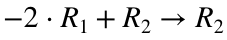.
Next, we will eliminate thecoefficient from the third equation. We can do this by multiplying the first equation by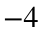and adding it to the third equation. We obtainThis step can be written as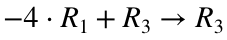.
Finally, we eliminate the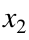coefficient from the third equation. We can do this by multiplying the second equation by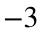and adding it to the third equation. (Note that it would not be a good idea to multiply the first equation byand add it to the third equation, because that would undo the work that we did in the last step.) We getThis step can be written as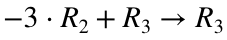.
Now our system is in upper triangular form, so we can use back substitution to solve it. The third equation is easy to solve and gives us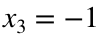. Plugging this into the second equation, we find that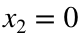. Plugging each of these into the first equation, we find that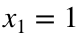.
The order in which we eliminated the coefficients is somewhat arbitrary, but it is important to be consistent so that we can turn this into code. (In addition, this particular order will make our life easier in the next section.) When we use this order (i.e., eliminate all of thecoefficients, then all of thecoefficients, etc.) the method is called Gaussian elimination. Hopefully you can see how this generalizes to larger systems. If you have trouble visualizing it, I encourage you to try experimenting with a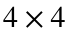system until it becomes clear. That said, the amount of arithmetic and writing rapidly becomes cumbersome as the number of equations and variables increases, so I will never ask you to solve anything larger than asystem by hand. Fortunately for us, lots of arithmetic in a prescribed order is exactly what computers are good at.
There is one problem with our method. To see what can go wrong, consider the systemThis is the same as one of the systems we solved above with back substitution except that the equations are in a different order, so we know that there is a solution. In fact, we know that the solution isand. However, the system is not upper triangular, and we cannot apply our method because we cannot eliminate the coefficient for x in the second equation without dividing by zero. Of course, we can just reorder our equations to solve this issue.
It turns out (although we won't prove it here) that this is the only possible obstacle. As long as our system of equations has a unique solution, we can always reorder our equations in a way that lets us use Gaussian elimination.

### Matrix notation

MATLAB has many builtin methods to solve systems of equations, but we first need to input our equations in a way that MATLAB will understand. To do so, we will rewrite our systems as matrix equations. We do this by putting all of the coefficients from our system in a matrix, putting all of the variables into a vector and putting all of the right-hand side values into another vector. For the first system, we have,and.
Now it will become apparent why we defined matrix multiplication the way we did. Since A is amatrix and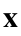is a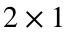vector, it makes sense to multiply the two together. (That is, the number of columns of A is the same as the number of rows of. This is as it should be, because A has one column for every variable in our equations andhas one row for every variable in our equations.) When we multiply these two, we should get avector (the number of rows of A by the number of columns of). Using the dot product definition, we getBut we know from the original equations that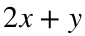is 5 and that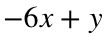is -3, so we haveThat is, we can rewrite our whole system as the single matrix equation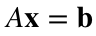.
Similarly, we can rewrite thesystem from above as the matrix equation, where,and.

### Solving systems in MATLAB

MATLAB has several useful builtin functions for solving systems of equations. All of these functions are designed to work with the matrix notation that we described in the previous section. To enter a system of equations into MATLAB, we therefore need to enter the matrix A and the vector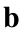. For example, we could define thesystem from above by writing
A = [2 1; -6 1];
b = [5; -3];
Notice that we did not explicitly write out the vector [x; y]. We will never tell MATLAB the names of our unknown variables, but all of the builtin solvers assume that we are solving for an unknown vector such that A times that vector equals.
The easiest way to solve a linear system in MATLAB is called the backslash (\) operator. We can solve the above system and save our answer in a vector x by writing
x = A\b
x = 2×1
1 3
Note that we never explicitly told MATLAB the names of our variables, but the order is implicitly represented by our equations. In particular, the coefficients in A are always in order. The coefficients in the first column of A correspond to the first variable, and the coefficients in the second column of A correspond to the second variable. Since we saved our solution in a vector named x, you can refer to these solutions in MATLAB as x(1) and x(2), but it is up to you to remember that x(1) corresponds to the variable x and x(2) corresponds to the variable y.
Similarly, we can enter theexample with
A = [2 1 1; 4 3 3; 8 7 9];
b = [1; 1; -1];
We can then solve this system with the command
x = A\b
x = 3×1
1 0 -1
The backslash operator is actually quite complicated because it uses specialized methods for different types of linear systems. For our purposes, though, we will assume that the code A\b uses one of two methods:
• If A is (upper or lower) triangular, then the backslash operator uses (back or forward) substitution to solve.
• If A is not triangular, then the backslash operator uses Gaussian elimination to solve, possibly after reordering the equations.

### Existence and uniqueness

As you already know from high school algebra, not all systems of equations have a solution, and if they do have a solution it is not necessarily unique. For example, the following system of equations does not have any solutions:because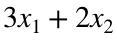cannot be both 5 and 4 simultaneously. However, the systemhas infinitely many solutions of the form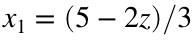and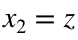. We won't prove it in this class, but one can show that these are the only three possibilities for any system of equations: Either the system has exactly one solution, or it has no solutions, or it has infinitely many solutions. Moreover, one can determine whether or not a system has exactly one solution just by looking at the left hand side (i.e., the matrix A), not the right hand side (i.e., the vector).
We will primarily be interested in solving systems that have a unique solution. If the system has infinitely many solutions, then you need more context to decide which solution is best. Similarly, if there are no solutions then you might be willing to accept something that is "close to" a solution, but you would need more context to decide what "close to" means. We will discuss the latter situation when we talk about data fitting, but for now we will only worry about solving systems that have a unique solution.
It is worth noting that MATLAB will usually give you some sort of answer when you use the backslash operator, but that answer may or may not be sensible. For instance, if we try to solve the above two sytems we get:
A = [3 2; 3 2];
b = [5; 4];
x = A\b
Warning: Matrix is singular to working precision.
x = 2×1
Inf -Inf
A = [3 2; 3 2];
b = [5; 5];
x = A\b
Warning: Matrix is singular to working precision.
x = 2×1
NaN NaN
In this case it is fairly obvious that these answers are not correct (although you do have to actually look at the entries of x to tell), but it is entirely possible for the backslash operator to give answers that do not involve strange values like Inf or NaN. Moreover, the backslash operator can be quite slow. (We will see exactly how slow in the next lecture.) Because of this, we don't want to go through all the work of solving a system only to find out that it doesn't actually have a solution. Fortunately, there are a few builtin functions in MATLAB that can tell you if a system has a solution. Three of these operators are (in order of usefulness) cond, rcond and det.
The function cond calculates the condition number of a matrix. We won't worry about the technical definition of the condition number, but the relevant feature for us is that the systemhas a unique solution if and only if the condition number of A is finite. If the condition number of A is infinite, then the system has either no solutions or infinitely many solutions. Calculating the condition number perfectly is not always possible, so cond only gives an approximation. In particular, it might not give actual infinity even if the system does not have a solution. If A has a very large condition number then you should assume that there is no unique solution. (We won't worry about exactly what "very large" means. You can safely assume that condition numbers larger than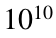are very large.)
For example,
A = [3 2; 3 2];
cond(A)
ans = 3.5394e+16
B = [2 1; -6 1];
cond(B)
ans = 5.0521
The function rcond calculates the reciprocal of the condition number of a matrix. That is, it calculates 1 / cond(A). This means that if rcond(A) is very close to zero then the system does not have a unique solution. rcond uses a different approximation method than cond. The rcond function tends to be faster, but produce a less accurate approximation of the condition number.
For example,
rcond(A)
ans = 0
rcond(B)
ans = 0.1429
Finally, the function det calculates the determinant of a matrix. If you ever take a more theoretical linear algebra class, you will spend a lot of time discussing the determinant, so it might come as a surprise to find that we will barely mention it in this class. It turns out that the determinant is very useful theoretically, but very inefficient to calculate. It does have the following useful property: A square system (that is, when you have the same number of equations as variables) has exactly one solution if and only if the determinant of A is non-zero. Unfortunately, the determinant is not defined for non-square matrices.
For example,
det(A)
ans = 0
det(B)
ans = 8
In general, if someone asks you to solve an equation, you should first check the condition number of A. If cond(A) is very large, then the correct answer is not x = A\b. Instead, the correct answer is to say "no."